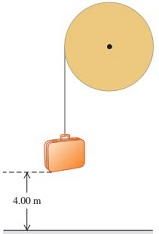# A light rope is wrapped several times around a lar A light rope is wrapped several times around a large wheel with a radius of 0.365 m . The wheel rotates in frictionless bearings about a stationary horizontal axis, as shown in the figure (Figure 1) . The free end of the rope is tied to a suitcase with a mass of 15.5 kg . The suitcase is released from rest at a height of 4.00 m above the ground. The suitcase has a speed of 3.90 m/s when it reaches the ground. Part A Calculate the angular velocity of the wheel when the suitcase reaches the ground. Express your answer in radians per second to three significant figures. Part B Calculate the moment of inertia of the wheel. Express your answer in kilogram meters squared to three significant figures

Question-AnswerCategory: Engineering MechanicsA light rope is wrapped several times around a lar A light rope is wrapped several times around a large wheel with a radius of 0.365 m . The wheel rotates in frictionless bearings about a stationary horizontal axis, as shown in the figure (Figure 1) . The free end of the rope is tied to a suitcase with a mass of 15.5 kg . The suitcase is released from rest at a height of 4.00 m above the ground. The suitcase has a speed of 3.90 m/s when it reaches the ground. Part A Calculate the angular velocity of the wheel when the suitcase reaches the ground. Express your answer in radians per second to three significant figures. Part B Calculate the moment of inertia of the wheel. Express your answer in kilogram meters squared to three significant figuresA light rope is wrapped several times around a large wheel with a radius of 0.365 m . The wheel rotates in frictionless bearings about a stationary horizontal axis, as shown in the figure (Figure 1) . The free end of the rope is tied to a suitcase with a mass of 15.5 kg . The suitcase is released from rest at a height of 4.00 m above the ground. The suitcase has a speed of 3.90 m/s when it reaches the ground.
Part A Calculate the angular velocity of the wheel when the suitcase reaches the ground. Express your answer in radians per second to three significant figures.
Part B Calculate the moment of inertia of the wheel. Express your answer in kilogram meters squared to three significant figures

Given,
mass = 15.5 kg
height = 4 m
speed = 3.9 m/s